傅里叶频谱_傅里叶级数和傅里叶变换都可以画出幅度频谱 - CSDN
• 1.图像的傅里叶频谱的意义 之前的博文其实已经归纳过这方面的内容了。我们常用的图像平滑处理，其实就是一个低通滤波，一定程度上去除高频信号，可以使得图像变得柔和（也就是平滑）。但是，在去除周期性噪声时候，...

1.图像的傅里叶频谱的意义

之前的博文其实已经归纳过这方面的内容了。我们常用的图像平滑处理，其实就是一个低通滤波，一定程度上去除高频信号，可以使得图像变得柔和（也就是平滑）。但是，在去除周期性噪声时候，空间域内的滤波（卷积）就不是那么好操作了。所以，这里时候，无论是理解起来方便，还是其他原因，都需要在频域内进行滤波。
详细的叙述还是在下面的博文里面啦！！！！

2. 傅里叶频谱的计算

这部分的内容，主要就是使用OpenCV自带的函数 void cvDFT( const CvArr* src, CvArr* dst, int flags, int nonzero_rows=0 )
去求取图像的傅里叶变换。这里，输出结果CvArr* dst由两个通道组成，分别代表了实部与虚部。我们再根据如下算式，就可以得到傅里叶频谱了。 |F(u,v)|=R2(u,v)+F2(u,v)‾‾‾‾‾‾‾‾‾‾‾‾‾‾‾‾‾√2
我自己也参考了很多人的代码，然后实现的代码如下。
IplImage* fft2(IplImage* image_input)
{
int dftWidth  = getOptimalDFTSize(image_input->width);
int dftHeight = getOptimalDFTSize(image_input->height);

//cout<< " Width" <<  image_input->width << "    " <<  dftWidth  << "\n";
//cout<< "Height" << image_input->height << "    " <<  dftHeight << "\n";

IPL_DEPTH_8U,
1);

IplImage *image_Re =0 , *image_Im = 0, *image_Fourier = 0;

image_Re = cvCreateImage(cvSize(dftWidth,dftHeight),IPL_DEPTH_64F,1);
image_Im = cvCreateImage(cvSize(dftWidth,dftHeight),IPL_DEPTH_64F,1);
image_Fourier = cvCreateImage(cvSize(dftWidth,dftHeight),IPL_DEPTH_64F,2);

//image_Im <--- 0
cvZero(image_Im);
//image_Fourier <--- image_Re
//image_Fourier <--- image_Im
cvMerge(image_Re,image_Im,0,0,image_Fourier);

cvDFT(image_Fourier,image_Fourier,CV_DXT_FORWARD);

//image_Fourier ---> image_Re
//image_Fourier ---> image_Im
cvSplit(image_Fourier,image_Re,image_Im,0,0);

//Mag = sqrt(Re^2 + Im^2)
cvPow(image_Re,image_Re,2.0);
cvPow(image_Im,image_Im,2.0);
cvPow(image_Re,image_Re,0.5);

// log (1 + Mag)
cvLog (image_Re,image_Re);

//  |-----|-----|           |-----|-----|
//  |  1  |  3  |           |  4  |  2  |
//  |-----|-----|   --->    |-----|-----|
//  |  2  |  4  |           |  3  |  1  |
//  |-----|-----|           |-----|-----|

IplImage *Fourier = cvCreateImage(cvSize(dftWidth,dftHeight),IPL_DEPTH_64F,1);
cvZero(image_Fourier);

int cx = image_Re->width/2;
int cy = image_Re->height/2;

cvSetImageROI(image_Re,cvRect( 0, 0,cx,cy));  // 1
cvSetImageROI( Fourier,cvRect(cx,cy,cx,cy));  // 4

cvSetImageROI(image_Re,cvRect(cx,cy,cx,cy));  // 4
cvSetImageROI( Fourier,cvRect( 0, 0,cx,cy));  // 1

cvSetImageROI(image_Re,cvRect(cx, 0,cx,cy));  // 3
cvSetImageROI( Fourier,cvRect( 0,cy,cx,cy));  // 2

cvSetImageROI(image_Re,cvRect( 0,cy,cx,cy));  // 2
cvSetImageROI( Fourier,cvRect(cx, 0,cx,cy));  // 3

cvResetImageROI(image_Re);
cvResetImageROI( Fourier);

cvNormalize(Fourier,Fourier,1,0,CV_C,NULL);

return(Fourier);
}123456789101112131415161718192021222324252627282930313233343536373839404142434445464748495051525354555657585960616263646566676869707172737475767778123456789101112131415161718192021222324252627282930313233343536373839404142434445464748495051525354555657585960616263646566676869707172737475767778
从这里开始，还是简单的分析一下代码吧。
int dftWidth  = getOptimalDFTSize(image_input->width);
int dftHeight = getOptimalDFTSize(image_input->height);
IPL_DEPTH_8U,
1);
cvCopyMakeBorder( image_input, image_padded, cvPoint(0,0), IPL_BORDER_CONSTANT,cvScalarAll(0)); 123456123456
这里参考了文献中的说法，在尺寸数为2,3,5的倍数的场合，计算的速度是最快的。所以使用函数getOptimalDFTSize()来寻找最匹配的尺寸，然后再同伙cvCopyMakeBorder()进行多余部分的填充，这里选的配置是将图放在从点(0,0)开始的位置，其余不足的地方，用0进行填充。
IplImage *image_Re =0 , *image_Im = 0, *image_Fourier = 0;

image_Re = cvCreateImage(cvSize(dftWidth,dftHeight),IPL_DEPTH_64F,1);
image_Im = cvCreateImage(cvSize(dftWidth,dftHeight),IPL_DEPTH_64F,1);
image_Fourier = cvCreateImage(cvSize(dftWidth,dftHeight),IPL_DEPTH_64F,2);

//image_Im <--- 0
cvZero(image_Im);
//image_Fourier <--- image_Re
//image_Fourier <--- image_Im
cvMerge(image_Re,image_Im,0,0,image_Fourier);

cvDFT(image_Fourier,image_Fourier,CV_DXT_FORWARD);

//image_Fourier ---> image_Re
//image_Fourier ---> image_Im
cvSplit(image_Fourier,image_Re,image_Im,0,0);1234567891011121314151617181912345678910111213141516171819
其实这里的很好理解的，将填充到最适尺寸的图像赋值给image_Re，将image_Im赋值为0。让后将这两层图复制到image_Fourier的两个通道里，然后使用函数cvDFT()进行傅里叶变换。得到结果还是存在于image_Fourier的两个通道里，分别代表实部与虚部，然后通过cvSplit()将其抽出到image_Re与image_Im里。
//Mag = sqrt(Re^2 + Im^2)
cvPow(image_Re,image_Re,2.0);
cvPow(image_Im,image_Im,2.0);
cvPow(image_Re,image_Re,0.5);

// log (1 + Mag)
cvLog (image_Re,image_Re); 123456789123456789
以上代码，实现了以下计算。 |F(u,v)|=R2(u,v)+F2(u,v)‾‾‾‾‾‾‾‾‾‾‾‾‾‾‾‾‾√2
还有就是进行了一个对数变换，这个也没的说，看傅里叶频谱的标配操作。
//  |-----|-----|           |-----|-----|
//  |  1  |  3  |           |  4  |  2  |
//  |-----|-----|   --->    |-----|-----|
//  |  2  |  4  |           |  3  |  1  |
//  |-----|-----|           |-----|-----|

IplImage *Fourier = cvCreateImage(cvSize(dftWidth,dftHeight),IPL_DEPTH_64F,1);
cvZero(image_Fourier);

int cx = image_Re->width/2;
int cy = image_Re->height/2;

cvSetImageROI(image_Re,cvRect( 0, 0,cx,cy));  // 1
cvSetImageROI( Fourier,cvRect(cx,cy,cx,cy));  // 4

cvSetImageROI(image_Re,cvRect(cx,cy,cx,cy));  // 4
cvSetImageROI( Fourier,cvRect( 0, 0,cx,cy));  // 1

cvSetImageROI(image_Re,cvRect(cx, 0,cx,cy));  // 3
cvSetImageROI( Fourier,cvRect( 0,cy,cx,cy));  // 2

cvSetImageROI(image_Re,cvRect( 0,cy,cx,cy));  // 2
cvSetImageROI( Fourier,cvRect(cx, 0,cx,cy));  // 3

cvResetImageROI(image_Re);
cvResetImageROI( Fourier);

cvNormalize(Fourier,Fourier,1,0,CV_C,NULL);

return(Fourier);1234567891011121314151617181920212223242526272829303132333412345678910111213141516171819202122232425262728293031323334
其实重头戏在这里，这里需要一个交换操作。至于为何所求得的傅里叶频谱为什么需要交换的原因是，这个代码求得的结果其实是范围[0,2π]内的傅里叶变换。而我们所需要的是[−π,π]内的结果。详细的原因，还是在我以前的博客里有说明。 [数字图像处理]频域滤波(1)–基础与低通滤波器

这里，我使用了ROI操作与cvAddWeighted()函数进行了实现。其运行的结果如下所示。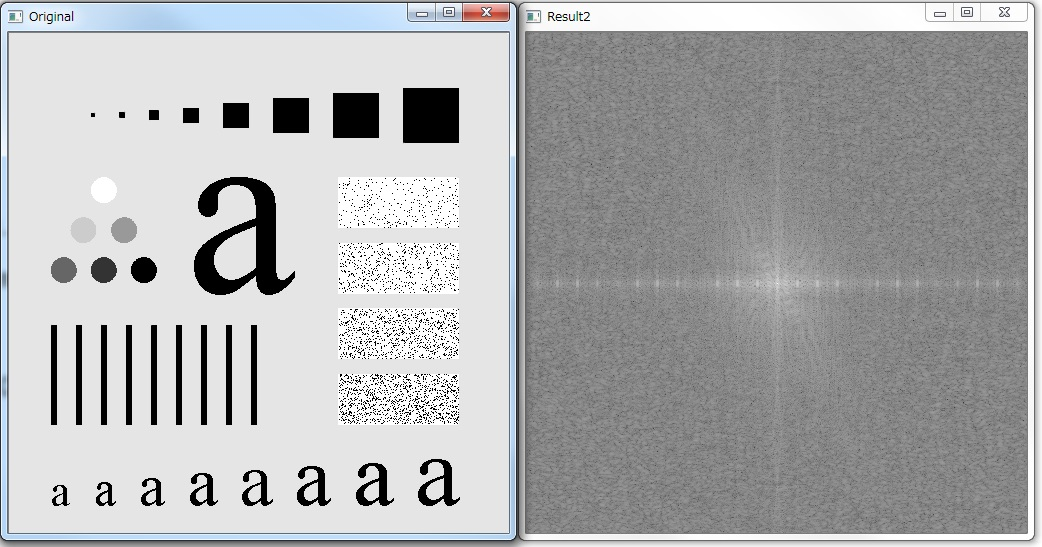恩，基本可以看出来，直流分量也被我移动到了中心，以上代码实现了傅里叶频谱的计算与显示。

3. 不用交换操作的代码

使用MATLAB去求取尺寸为M×N图像f的傅里叶频谱时候，通常会用fft2(f,2*M,2*N)。使用此函数求得的福利叶变换，其实还是[0,2π]范围内的傅里叶变换。要想使得傅里叶频谱的DC分量位于中心的话，其实还是需要一些别的操作的。冈萨雷斯的《数字图像处理》一书中，对于这个问题，是利用了傅里叶变换的评议特性，即 f(x,y)=f(x,y)×(−1)x+y
然后再对函数f(x,y)进行福利叶变换，所得到结果，就是所需要的是[−π,π]内傅里叶变换的结果。具体的原理与推导，还是参看冈萨雷斯的《数字图像处理》英文原本的p258页左右，中文译本的p148左右。当然，嫌麻烦的话，还可以看我的博文，博文中也简明推导了平移特性。 [数字图像处理]频域滤波(1)–基础与低通滤波器
为此，实现的代码变为了如下形式。
IplImage* fft2_New(IplImage* image_input)
{
int dftWidth  = getOptimalDFTSize(image_input->width);
int dftHeight = getOptimalDFTSize(image_input->height);

cout<< " Width" <<  image_input->width << "    " <<  dftWidth  << "\n";
cout<< "Height" << image_input->height << "    " <<  dftHeight << "\n";

IPL_DEPTH_8U,
1);

IplImage *image_Re =0 , *image_Im = 0, *image_Fourier = 0;

image_Re = cvCreateImage(cvSize(dftWidth,dftHeight),IPL_DEPTH_64F,1);
image_Im = cvCreateImage(cvSize(dftWidth,dftHeight),IPL_DEPTH_64F,1);
image_Fourier = cvCreateImage(cvSize(dftWidth,dftHeight),IPL_DEPTH_64F,2);

double pixel;
{
{
pixel = ((x+y)%2 == 0)?(pixel):((-1)*pixel);
cvSetReal2D(image_Re,x,y,pixel);
}
}

//image_Im <--- 0
cvZero(image_Im);
//image_Fourier <--- image_Re
//image_Fourier <--- image_Im
cvMerge(image_Re,image_Im,0,0,image_Fourier);

cvDFT(image_Fourier,image_Fourier,CV_DXT_FORWARD);

//image_Fourier ---> image_Re
//image_Fourier ---> image_Im
cvSplit(image_Fourier,image_Re,image_Im,0,0);

//Mag = sqrt(Re^2 + Im^2)
cvPow(image_Re,image_Re,2.0);
cvPow(image_Im,image_Im,2.0);
cvPow(image_Re,image_Re,0.5);

// log (1 + Mag)
cvLog (image_Re,image_Re);

cvNormalize(image_Re,image_Re,1,0,CV_C,NULL);

return(image_Re);
}123456789101112131415161718192021222324252627282930313233343536373839404142434445464748495051525354555657123456789101112131415161718192021222324252627282930313233343536373839404142434445464748495051525354555657
在这里，由于考虑到计算的原因，我将f(x,y)=f(x,y)×(−1)x+y这个计算，用下面代码去进行了实现。
for(int y=0;y<image_padded->height;y++)
{
{
pixel = ((x+y)%2 == 0)?(pixel):((-1)*pixel);
cvSetReal2D(image_Re,x,y,pixel);
}
}123456789123456789
其实也就相当于，x+y是偶数的话f(x,y)不变，x+y是奇数的话f(x,y)变为负。用这样一个简单的判断去实现。其运行的结果，如下所示。

原图的傅里叶频谱使用11×11高斯滤波器后的傅里叶频谱从实验结果看来，可以看出以下两点
对于原图而言两个函数的结果基本一致，两个函数都得到了正确的结果。使用平滑处理后，频谱的高频成分明显变小，对于空间域的图像而言，图像变得模糊了。


展开全文图像 傅里叶
• 文章目录一、傅里叶变换后的频谱对应图像为什么四角亮?二、将零点移到中心的原因三、高频和低频概念理解 一、傅里叶变换后的频谱对应图像为什么四角亮? >> f = imread('fft2.tif'); >> F = fft2(f); ...
文章目录一、傅里叶变换后的频谱对应图像为什么四角亮?二、将零点移到中心的原因三、高频和低频概念理解
一、傅里叶变换后的频谱对应图像为什么四角亮?
>> f = imread('fft2.tif');
>> F = fft2(f);	% 得到图像 f 的傅里叶变换算法
>> S = abs(F);	% 获得傅里叶谱
>> imshow(S)
>> imshow(f),figure,imshow(S,[])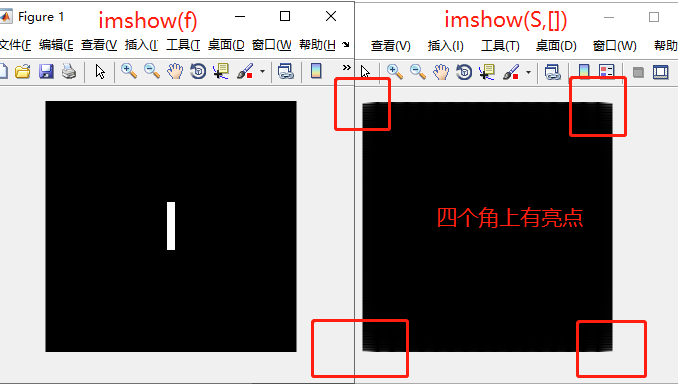解答：
二维离散傅里叶变换结果中频率成分分布示意图：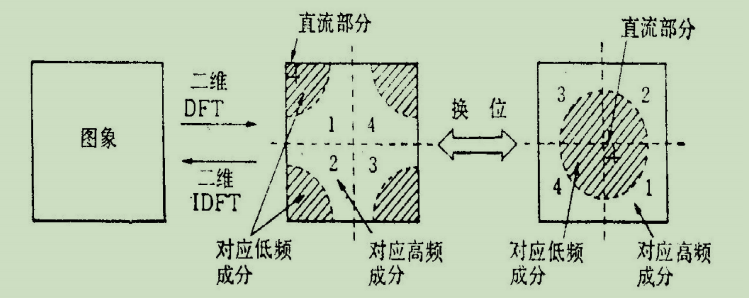所以因为变换后的四角位置刚好对应着图像的低频成分，而一般来说图像的能量都集中在低频分量上，因此变换后低频位置处的幅度会大些，显示出来就更亮了
二、将零点移到中心的原因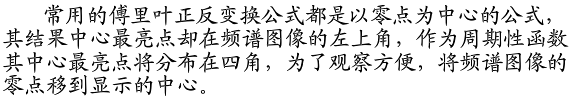三、高频和低频概念理解
参考 1：烈日烤鱼
比如有如下数组
{1, 10, 1, 10, 1, 10, 1, 10, 1, 10, 1, 10}和{1, 2, 3, 4, 5, 4, 3, 2, 1, 2, 3, 4, 5, 4, 3, 2, 1,}
你直观的理解下,哪个数组的"频率"大点?
你可以用纵坐标代表数组值,横坐标代表数组index描点看看,显然是第一个数组的频率高.
所以对于数组来说,数字之间变化剧烈,代表高频,柔和代表低频.
同理,对于图像来说,那就是灰度变化快的是高频,慢的是低频.比如一个物体的边缘,就是高频信号,物体内部,就是低频.
而傅立叶变换无非是告诉你这副图像上XXX频率的信号有多少多少, YYY的频率有多少多少
那换句话说就是,图像的傅立叶变换可以让你直观的看到这幅图总体上"剧烈"的变化有多少,"柔和"的变化有多少
一副整体很模糊的图,傅立叶变换后显示的低频分量就很多
一副整体灰度变化很剧烈的图,傅立叶变换后显示的高频分量就很多
同理,如果你在频域上将高频分量去掉,再反变换回去,那图片就会变的模糊.
参考 2：技术先生
亮度或灰度变化激烈的地方对应高频成分，如边缘；变化不大的地方对于低频成分，如大片色块区画个直方图，大块区域是低频，小块或离散的是高频把图像看成二维函数，变化剧烈的地方就对应高频，反之低频。
例如：
一幅图象，你戴上眼镜，盯紧了一个地方看到的是高频分量
摘掉眼镜，眯起眼睛，模模糊糊看到的就是低频分量。
图像的高低频是对图像各个位置之间强度变化的一种度量方法.
低频分量:主要对整副图像的强度的综合度量.
高频分量:主要是对图像边缘和轮廓的度量.
如果一副图像的各个位置的强度大小相等,则图像只存在低频分量,从图像的频谱图上看,只有一个主峰,且位于频率为零的位置.
如果一副图像的各个位置的强度变化剧烈,则图像不仅存在低频分量,同时也存在多种高频分量,从图像的频谱上看,不仅有一个主峰,同时也存在多个旁峰.


展开全文• **傅里叶变换** 对图像的傅里叶变换，就是将图像从图像空间变换到频率空间，从而可以在频率域对图像进行处理。 1、傅里叶变换及其反变换 在numpy中自带了函数fft2进行二维傅里叶变换，它其实是离散快速傅里叶变换。...
								**傅里叶变换**

对图像的傅里叶变换，就是将图像从图像空间变换到频率空间，从而可以在频率域对图像进行处理。
1、傅里叶变换及其反变换
在numpy中自带了函数fft2进行二维傅里叶变换，它其实是离散快速傅里叶变换。
在频率域对图像进行处理后，要将其反变换到空间域才能显示图像。
可用函数 np.fft.ifft2（）函数进行傅里叶反变换。

import cv2 as cv
import numpy as np
from matplotlib import pyplot as plt
import matplotlib as mpl

mpl.rcParams['font.sans-serif']  = ['SimHei']	# 指定字体，不然会乱码
f = np.fft.fft2(img)	# 将空间域转化为频率域
mf = np.log(np.abs(f))	# 取绝对值f 对数

# 傅里叶反变换
fan = np.fft.ifft2(f)
mfan = np.abs(fan)

plt.subplot(131),plt.imshow(img, cmap='gray'),plt.title('原图')
plt.subplot(132),plt.imshow(mf, cmap='gray'),plt.title('傅里叶频谱')
plt.subplot(133),plt.imshow(mfan, cmap='gray'),plt.title('傅里叶反变换')

plt.show()


如下图，左图是lena图像，中间图是它的傅里叶频谱图，最后一张即是反变换回来的图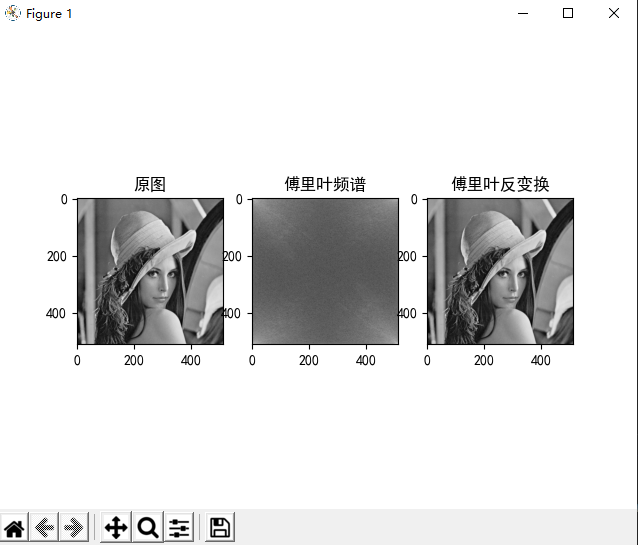2.将低频移至中心
在此傅里叶频谱中，频率为0的分量在其左上角，但是一般情况下我们会将其移至中心，再对其进行处理。
可以用函数fftshift()将低频部分移至中心。

import cv2 as cv
import numpy as np
from matplotlib import pyplot as plt
import matplotlib as mpl

mpl.rcParams['font.sans-serif']  = ['SimHei']	# 指定字体，不然会乱码
f = np.fft.fft2(img)	# 将空间域转化为频率域
mf = np.log(np.abs(f))	# 取绝对值f 对数，mp为频谱图

# 将低频移至傅里叶频谱中心
fshift = np.fft.fftshift(f)
mfshift = np.log(np.abs(fshift))

plt.subplot(131),plt.imshow(img, cmap='gray'),plt.title('原图')
plt.subplot(132),plt.imshow(mf, cmap='gray'),plt.title('傅里叶频谱')
plt.subplot(133),plt.imshow(mfshift, cmap='gray'),plt.title('低频移至傅里叶频谱中心')

plt.show()


右图就是将低频移至中心的傅里叶频谱图。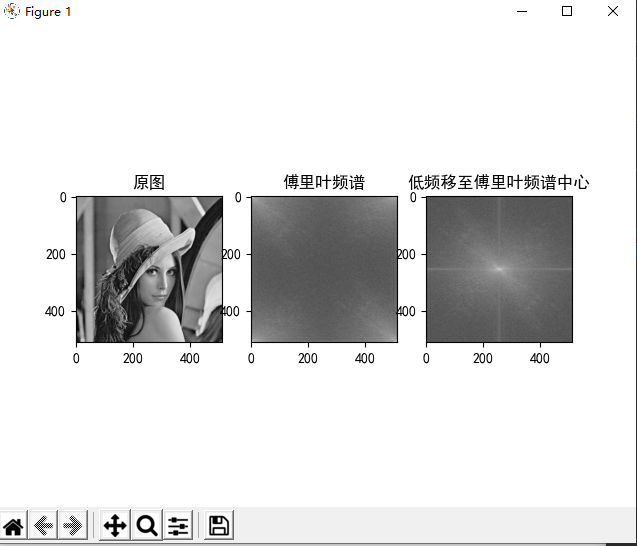展开全文opencv
• 要让读者在不看任何数学公式的情况下理解傅里叶分析。 傅里叶分析不仅仅是一个数学工具，更是一种可以彻底颠覆一个人以前世界观的思维模式。但不幸的是，傅里叶分析的公式看起来太复杂了，所以很多大一新生上来就懵...


这篇文章的核心思想就是：
要让读者在不看任何数学公式的情况下理解傅里叶。
傅里叶分析不仅仅是一个数学工具，更是一种可以彻底颠覆一个人以前世界观的思维模式。但不幸的是，傅里叶分析的公式看起来太复杂了，所以很多大一新生上来就懵圈并从此对它深恶痛绝。老实说，这么有意思的东西居然成了大学里的杀手课程，不得不归咎于编教材的人实在是太严肃了。（您把教材写得好玩一点会死吗？会死吗？）所以我一直想写一个有意思的文章来解释傅里叶分析，有可能的话高中生都能看懂的那种。所以，不管读到这里的您从事何种工作，我保证您都能看懂，并且一定将体会到通过傅里叶分析看到世界另一个样子时的快感。至于对于已经有一定基础的朋友，也希望不要看到会的地方就急忙往后翻，仔细读一定会有新的发现。
————以上是定场诗————
下面进入正题：
抱歉，还是要啰嗦一句：其实学习本来就不是易事，我写这篇文章的初衷也是希望大家学习起来更加轻松，充满乐趣。但是千万！千万不要把这篇文章收藏起来，或是存下地址，心里想着：以后有时间再看。这样的例子太多了，也许几年后你都没有再打开这个页面。无论如何，耐下心，读下去。这篇文章要比读课本要轻松、开心得多……
p.s.本文无论是cos还是sin，都统一用“正弦波”（Sine Wave）一词来代表简谐波。
一、什么是频域
从我们出生，我们看到的世界都以时间贯穿，股票的走势、人的身高、汽车的轨迹都会随着时间发生改变。这种以时间作为参照来观察动态世界的方法我们称其为时域分析。而我们也想当然的认为，世间万物都在随着时间不停的改变，并且永远不会静止下来。但如果我告诉你，用另一种方法来观察世界的话，你会发现世界是永恒不变的，你会不会觉得我疯了？我没有疯，这个静止的世界就叫做频域。
先举一个公式上并非很恰当，但意义上再贴切不过的例子：
在你的理解中，一段音乐是什么呢？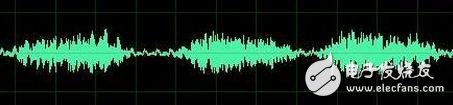这是我们对音乐最普遍的理解，一个随着时间变化的震动。但我相信对于乐器小能手们来说，音乐更直观的理解是这样的：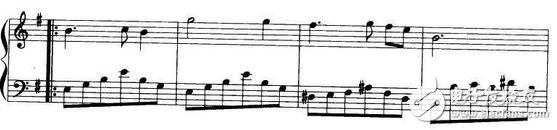好的！下课，同学们再见。

是的，其实这一段写到这里已经可以结束了。上图是音乐在时域的样子，而下图则是音乐在频域的样子。所以频域这一概念对大家都从不陌生，只是从来没意识到而已。
现在我们可以回过头来重新看看一开始那句痴人说梦般的话：世界是永恒的。
将以上两图简化：
时域：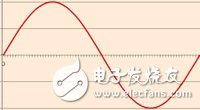频域：在时域，我们观察到钢琴的琴弦一会上一会下的摆动，就如同一支股票的走势；而在频域，只有那一个永恒的音符。
所以
你眼中看似落叶纷飞变化无常的世界，实际只是躺在上帝怀中一份早已谱好的乐章。
抱歉，这不是一句鸡汤文，而是黑板上确凿的公式：傅里叶同学告诉我们，任何周期函数，都可以看作是不同振幅，不同相位正弦波的叠加。在第一个例子里我们可以理解为，利用对不同琴键不同力度，不同时间点的敲击，可以组合出任何一首乐曲。
而贯穿时域与频域的方法之一，就是传中说的傅里叶分析。傅里叶分析可分为傅里叶级数（Fourier Serie）和傅里叶变换(Fourier Transformation)，我们从简单的开始谈起。
二、傅里叶级数(Fourier Series)的频谱
还是举个栗子并且有图有真相才好理解。
如果我说我能用前面说的正弦曲线波叠加出一个带90度角的矩形波来，你会相信吗？你不会，就像当年的我一样。但是看看下图：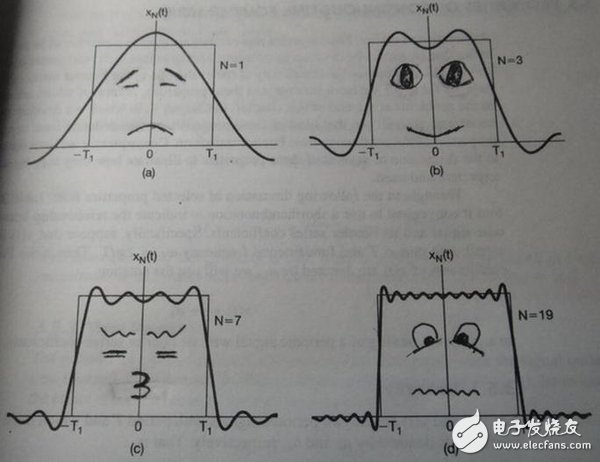第一幅图是一个郁闷的正弦波cos（x）
第二幅图是2个卖萌的正弦波的叠加cos(x)+a.cos(3x)
第三幅图是4个发春的正弦波的叠加
第四幅图是10个便秘的正弦波的叠加
随着正弦波数量逐渐的增长，他们最终会叠加成一个标准的矩形，大家从中体会到了什么道理？
（只要努力，弯的都能掰直！）
随着叠加的递增，所有正弦波中上升的部分逐渐让原本缓慢增加的曲线不断变陡，而所有正弦波中下降的部分又抵消了上升到最高处时继续上升的部分使其变为水平线。一个矩形就这么叠加而成了。但是要多少个正弦波叠加起来才能形成一个标准90度角的矩形波呢？不幸的告诉大家，答案是无穷多个。（上帝：我能让你们猜着我？）
不仅仅是矩形，你能想到的任何波形都是可以如此方法用正弦波叠加起来的。这是没
有接触过傅里叶分析的人在直觉上的第一个难点，但是一旦接受了这样的设定，游戏就开始有意思起来了。
还是上图的正弦波累加成矩形波，我们换一个角度来看看：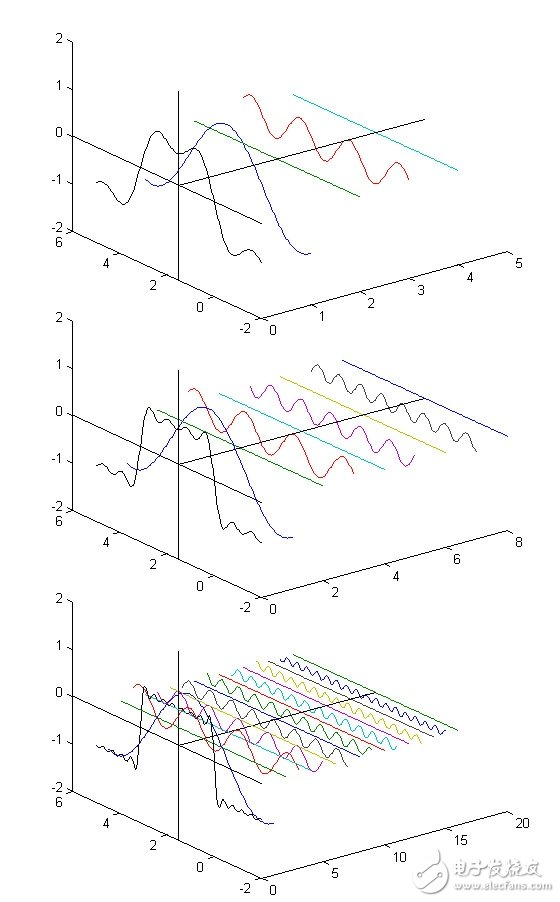在这几幅图中，最前面黑色的线就是所有正弦波叠加而成的总和，也就是越来越接近矩形波的那个图形。而后面依不同颜色排列而成的正弦波就是组合为矩形波的各个分量。这些正弦波按照频率从低到高从前向后排列开来，而每一个波的振幅都是不同的。一定有细心的读者发现了，每两个正弦波之间都还有一条直线，那并不是分割线，而是振幅为0的正弦波！也就是说，为了组成特殊的曲线，有些正弦波成分是不需要的。

这里，不同频率的正弦波我们成为频率分量。
好了，关键的地方来了！！
如果我们把第一个频率最低的频率分量看作“1”，我们就有了构建频域的最基本单元。
对于我们最常见的有理数轴，数字“1”就是有理数轴的基本单元。
时域的基本单元就是“1秒”，如果我们将一个角频率为的正弦波cos（t）看作基础，那么频域的基本单元就是。
有了“1”，还要有“0”才能构成世界，那么频域的“0”是什么呢？cos（0t）就是一个周期无限长的正弦波，也就是一条直线！所以在频域，0频率也被称为直流分量，在傅里叶级数的叠加中，它仅仅影响全部波形相对于数轴整体向上或是向下而不改变波的形状。
接下来，让我们回到初中，回忆一下已经死去的八戒，啊不，已经死去的老师是怎么定义正弦波的吧。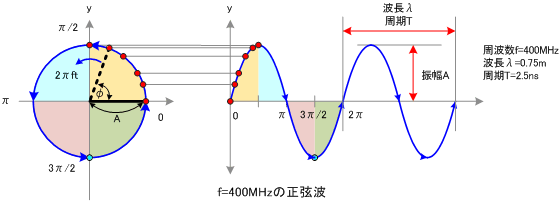正弦波就是一个圆周运动在一条直线上的投影。所以频域的基本单元也可以理解为一个始终在旋转的圆：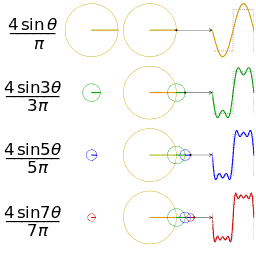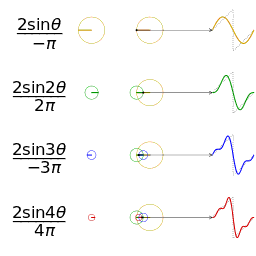介绍完了频域的基本组成单元，我们就可以看一看一个矩形波，在频域里的另一个模样了：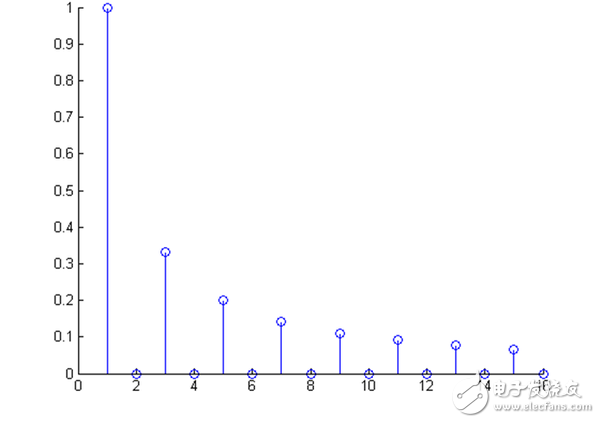这是什么奇怪的东西？

这就是矩形波在频域的样子，是不是完全认不出来了？教科书一般就给到这里然后留给了读者无穷的遐想，以及无穷的吐槽，其实教科书只要补一张图就足够了：频域图像，也就是俗称的频谱，就是——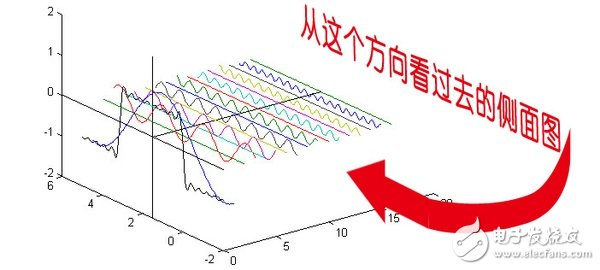再清楚一点：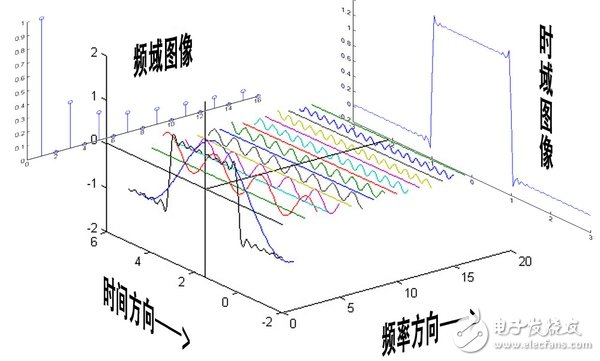可以发现，在频谱中，偶数项的振幅都是0，也就对应了图中的彩色直线。振幅为0的正弦波。老实说，在我学傅里叶变换时，维基的这个图还没有出现，那时我就想到了这种表达方法，而且，后面还会加入维基没有表示出来的另一个谱——相位谱。
但是在讲相位谱之前，我们先回顾一下刚刚的这个例子究竟意味着什么。记得前面说过的那句“世界是静止的”吗？估计好多人对这句话都已经吐槽半天了。想象一下，世界上每一个看似混乱的表象，实际都是一条时间轴上不规则的曲线，但实际这些曲线都是由这些无穷无尽的正弦波组成。我们看似不规律的事情反而是规律的正弦波在时域上的投影，而正弦波又是一个旋转的圆在直线上的投影。那么你的脑海中会产生一个什么画面呢？
我们眼中的世界就像皮影戏的大幕布，幕布的后面有无数的齿轮，大齿轮带动小齿轮，小齿轮再带动更小的。在最外面的小齿轮上有一个小人——那就是我们自己。我们只看到这个小人毫无规律的在幕布前表演，却无法预测他下一步会去哪。而幕布后面的齿轮却永远一直那样不停的旋转，永不停歇。这样说来有些宿命论的感觉。说实话，这种对人生的描绘是我一个朋友在我们都是高中生的时候感叹的，当时想想似懂非懂，直到有一天我学到了傅里叶级数……
三、傅里叶级数（Fourier Series）的相位谱
上一章的关键词是：从侧面看。这一章的关键词是：从下面看。
在这一章最开始，我想先回答很多人的一个问题：傅里叶分析究竟是干什么用的？这段相对比较枯燥，已经知道了的同学可以直接跳到下一个分割线。
先说一个最直接的用途。无论听广播还是看电视，我们一定对一个词不陌生——频道。频道频道，就是频率的通道，不同的频道就是将不同的频率作为一个通道来进行信息传输。下面大家尝试一件事：
先在纸上画一个sin（x），不一定标准，意思差不多就行。不是很难吧。
好，接下去画一个sin（3x）+sin（5x）的图形。
别说标准不标准了，曲线什么时候上升什么时候下降你都不一定画的对吧？
好，画不出来不要紧，我把sin（3x）+sin（5x）的曲线给你，但是前提是你不知道这个曲线的方程式，现在需要你把sin（5x）给我从图里拿出去，看看剩下的是什么。这基本是不可能做到的。
但是在频域呢？则简单的很，无非就是几条竖线而已。
所以很多在时域看似不可能做到的数学操作，在频域相反很容易。这就是需要傅里叶变换的地方。尤其是从某条曲线中去除一些特定的频率成分，这在工程上称为滤波，是信号处理最重要的概念之一，只有在频域才能轻松的做到。
再说一个更重要，但是稍微复杂一点的用途——求解微分方程。（这段有点难度，看不懂的可以直接跳过这段）微分方程的重要性不用我过多介绍了。各行各业都用的到。但是求解微分方程却是一件相当麻烦的事情。因为除了要计算加减乘除，还要计算微分积分。而傅里叶变换则可以让微分和积分在频域中变为乘法和除法，大学数学瞬间变小学算术有没有。
傅里叶分析当然还有其他更重要的用途，我们随着讲随着提。
————————————————————————————————————
下面我们继续说相位谱：
通过时域到频域的变换，我们得到了一个从侧面看的频谱，但是这个频谱并没有包含时域中全部的信息。因为频谱只代表每一个对应的正弦波的振幅是多少，而没有提到相位。基础的正弦波A.sin(wt+θ)中，振幅，频率，相位缺一不可，不同相位决定了波的位置，所以对于频域分析，仅仅有频谱（振幅谱）是不够的，我们还需要一个相位谱。那么这个相位谱在哪呢？我们看下图，这次为了避免图片太混论，我们用7个波叠加的图。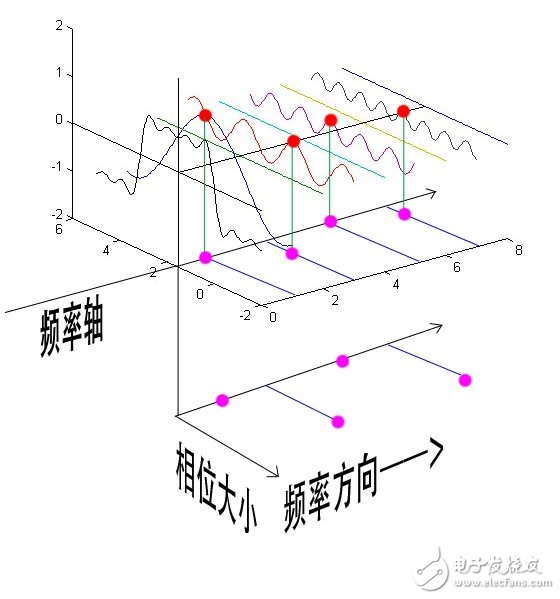鉴于正弦波是周期的，我们需要设定一个用来标记正弦波位置的东西。在图中就是那些小红点。小红点是距离频率轴最近的波峰，而这个波峰所处的位置离频率轴有多远呢？为了看的更清楚，我们将红色的点投影到下平面，投影点我们用粉色点来表示。当然，这些粉色的点只标注了波峰距离频率轴的距离，并不是相位。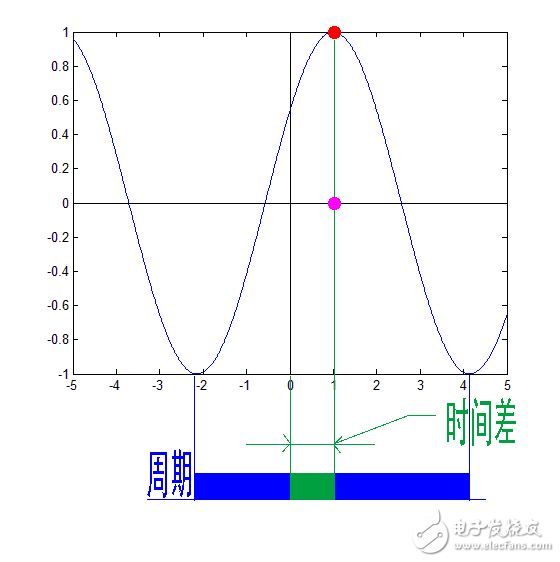这里需要纠正一个概念：时间差并不是相位差。如果将全部周期看作2Pi或者360度的话，相位差则是时间差在一个周期中所占的比例。我们将时间差除周期再乘2Pi，就得到了相位差。

在完整的立体图中，我们将投影得到的时间差依次除以所在频率的周期，就得到了最下面的相位谱。所以，频谱是从侧面看，相位谱是从下面看。下次偷看女生裙底被发现的话，可以告诉她：“对不起，我只是想看看你的相位谱。”
注意到，相位谱中的相位除了0，就是Pi。因为cos（t+Pi）=-cos（t），所以实际上相位为Pi的波只是上下翻转了而已。对于周期方波的傅里叶级数，这样的相位谱已经是很简单的了。另外值得注意的是，由于cos（t+2Pi）=cos（t），所以相位差是周期的，pi和3pi，5pi，7pi都是相同的相位。人为定义相位谱的值域为(-pi，pi]，所以图中的相位差均为Pi。

最后来一张大集合：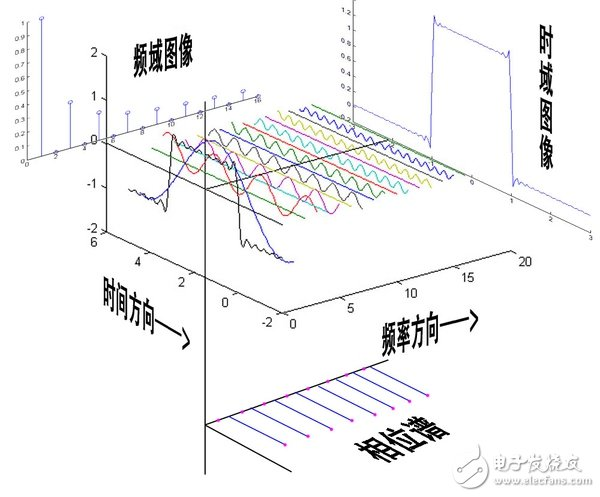四、傅里叶变换（Fourier Transformation）
相信通过前面三章，大家对频域以及傅里叶级数都有了一个全新的认识。但是文章在一开始关于钢琴琴谱的例子我曾说过，这个栗子是一个公式错误，但是概念典型的例子。所谓的公式错误在哪里呢？

傅里叶级数的本质是将一个周期的信号分解成无限多分开的（离散的）正弦波，但是宇宙似乎并不是周期的。曾经在学数字信号处理的时候写过一首打油诗：
往昔连续非周期，
回忆周期不连续，
任你ZT、DFT，
还原不回去。
（请无视我渣一样的文学水平……）
在这个世界上，有的事情一期一会，永不再来，并且时间始终不曾停息地将那些刻骨铭心的往昔连续的标记在时间点上。但是这些事情往往又成为了我们格外宝贵的回忆，在我们大脑里隔一段时间就会周期性的蹦出来一下，可惜这些回忆都是零散的片段，往往只有最幸福的回忆，而平淡的回忆则逐渐被我们忘却。因为，往昔是一个连续的非周期信号，而回忆是一个周期离散信号。
是否有一种数学工具将连续非周期信号变换为周期离散信号呢？抱歉，真没有。
比如傅里叶级数，在时域是一个周期且连续的函数，而在频域是一个非周期离散的函数。这句话比较绕嘴，实在看着费事可以干脆回忆第一章的图片。
而在我们接下去要讲的傅里叶变换，则是将一个时域非周期的连续信号，转换为一个在频域非周期的连续信号。
算了，还是上一张图方便大家理解吧：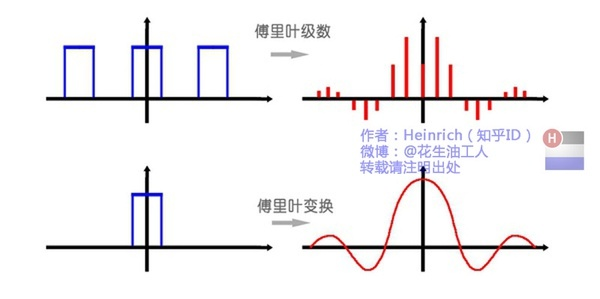或者我们也可以换一个角度理解：傅里叶变换实际上是对一个周期无限大的函数进行傅里叶变换。
所以说，钢琴谱其实并非一个连续的频谱，而是很多在时间上离散的频率，但是这样的一个贴切的比喻真的是很难找出第二个来了。
因此在傅里叶变换在频域上就从离散谱变成了连续谱。那么连续谱是什么样子呢？
你见过大海么？
为了方便大家对比，我们这次从另一个角度来看频谱，还是傅里叶级数中用到最多的那幅图，我们从频率较高的方向看。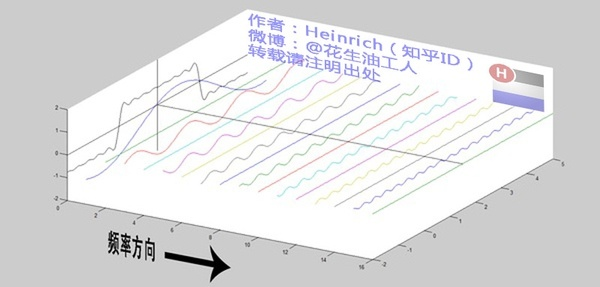以上是离散谱，那么连续谱是什么样子呢？
尽情的发挥你的想象，想象这些离散的正弦波离得越来越近，逐渐变得连续……
直到变得像波涛起伏的大海：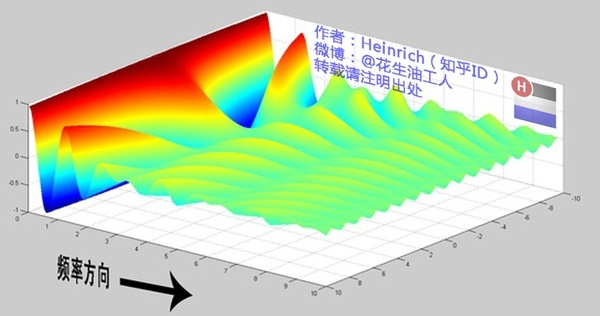很抱歉，为了能让这些波浪更清晰的看到，我没有选用正确的计算参数，而是选择了一些让图片更美观的参数，不然这图看起来就像屎一样了。
不过通过这样两幅图去比较，大家应该可以理解如何从离散谱变成了连续谱的了吧？原来离散谱的叠加，变成了连续谱的累积。所以在计算上也从求和符号变成了积分符号。
不过，这个故事还没有讲完，接下去，我保证让你看到一幅比上图更美丽壮观的图片，但是这里需要介绍到一个数学工具才能然故事继续，这个工具就是——
五、宇宙耍帅第一公式：欧拉公式
虚数i这个概念大家在高中就接触过，但那时我们只知道它是-1的平方根，可是它真正的意义是什么呢?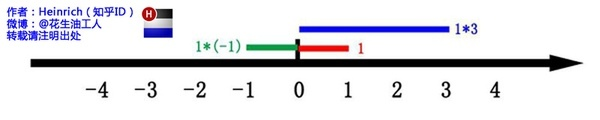这里有一条数轴，在数轴上有一个红色的线段，它的长度是1。当它乘以3的时候，它的长度发生了变化，变成了蓝色的线段，而当它乘以-1的时候，就变成了绿色的线段，或者说线段在数轴上围绕原点旋转了180度。

我们知道乘-1其实就是乘了两次 i使线段旋转了180度，那么乘一次 i 呢——答案很简单——旋转了90度。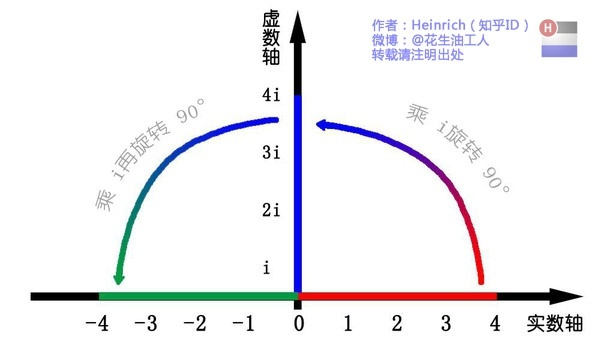同时，我们获得了一个垂直的虚数轴。实数轴与虚数轴共同构成了一个复数的平面，也称复平面。这样我们就了解到，乘虚数i的一个功能——旋转。
现在，就有请宇宙第一耍帅公式欧拉公式隆重登场——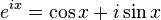这个公式在数学领域的意义要远大于傅里叶分析，但是乘它为宇宙第一耍帅公式是因为它的特殊形式——当x等于Pi的时候。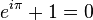经常有理工科的学生为了跟妹子表现自己的学术功底，用这个公式来给妹子解释数学之美：”石榴姐你看，这个公式里既有自然底数e，自然数1和0，虚数i还有圆周率pi，它是这么简洁，这么美丽啊！“但是姑娘们心里往往只有一句话：”臭屌丝……“
这个公式关键的作用，是将正弦波统一成了简单的指数形式。我们来看看图像上的涵义：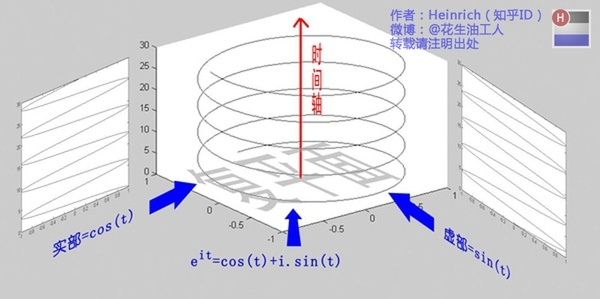欧拉公式所描绘的，是一个随着时间变化，在复平面上做圆周运动的点，随着时间的改变，在时间轴上就成了一条螺旋线。如果只看它的实数部分，也就是螺旋线在左侧的投影，就是一个最基础的余弦函数。而右侧的投影则是一个正弦函数。
关于复数更深的理解，大家可以参考：
复数的物理意义是什么？

这里不需要讲的太复杂，足够让大家理解后面的内容就可以了。
六、指数形式的傅里叶变换
有了欧拉公式的帮助，我们便知道：正弦波的叠加，也可以理解为螺旋线的叠加在实数空间的投影。而螺旋线的叠加如果用一个形象的栗子来理解是什么呢？
光波
高中时我们就学过，自然光是由不同颜色的光叠加而成的，而最著名的实验就是牛顿师傅的三棱镜实验：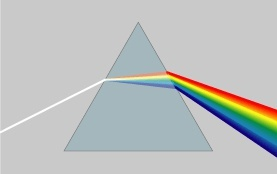所以其实我们在很早就接触到了光的频谱，只是并没有了解频谱更重要的意义。
但不同的是，傅里叶变换出来的频谱不仅仅是可见光这样频率范围有限的叠加，而是频率从0到无穷所有频率的组合。
这里，我们可以用两种方法来理解正弦波：
第一种前面已经讲过了，就是螺旋线在实轴的投影。
另一种需要借助欧拉公式的另一种形式去理解：将以上两式相加再除2，得到：这个式子可以怎么理解呢？
我们刚才讲过，e^(it)可以理解为一条逆时针旋转的螺旋线，那么e^(-it)则可以理解为一条顺时针旋转的螺旋线。而cos(t)则是这两条旋转方向不同的螺旋线叠加的一半，因为这两条螺旋线的虚数部分相互抵消掉了！
举个例子的话，就是极化方向不同的两束光波，磁场抵消，电场加倍。
这里，逆时针旋转的我们称为正频率，而顺时针旋转的我们称为负频率（注意不是复频率）。
好了，刚才我们已经看到了大海——连续的傅里叶变换频谱，现在想一想，连续的螺旋线会是什么样子：
想象一下再往下翻：
|
|

|

|

|

|

|

|

|

|

|

|

|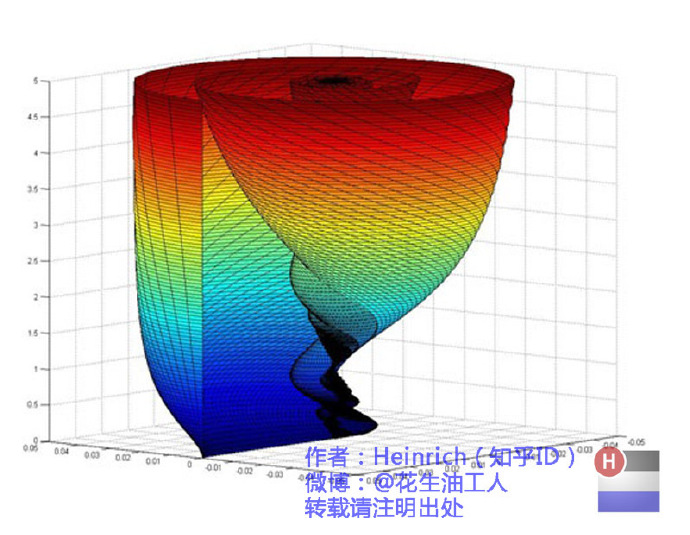是不是很漂亮？

你猜猜，这个图形在时域是什么样子？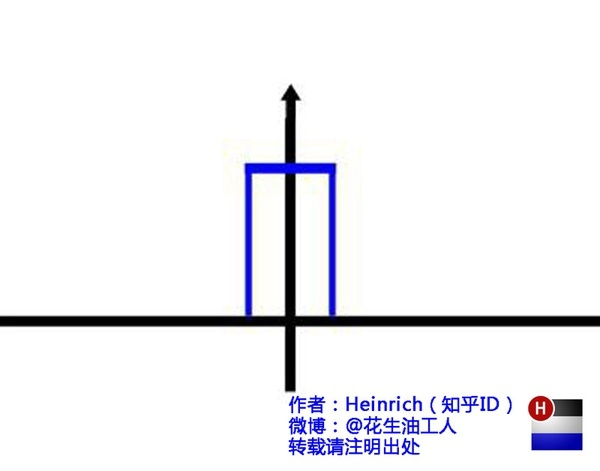哈哈，是不是觉得被狠狠扇了一个耳光。数学就是这么一个把简单的问题搞得很复杂的东西。

顺便说一句，那个像大海螺一样的图，为了方便观看，我仅仅展示了其中正频率的部分，负频率的部分没有显示出来。
如果你认真去看，海螺图上的每一条螺旋线都是可以清楚的看到的，每一条螺旋线都有着不同的振幅（旋转半径），频率（旋转周期）以及相位。而将所有螺旋线连成平面，就是这幅海螺图了。
好了，讲到这里，相信大家对傅里叶变换以及傅里叶级数都有了一个形象的理解了，我们最后用一张图来总结一下：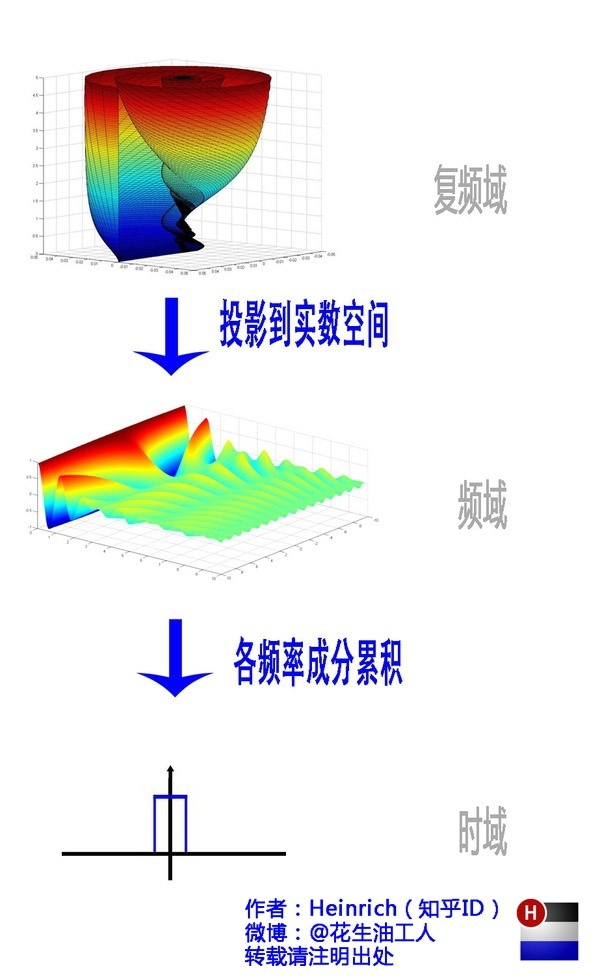老实说，数学工具对于工科生和对于理科生来说，意义是完全不同的。工科生只要理解了，会用，会查，就足够了。但是很多高校却将这些重要的数学课程教给数学系的老师去教。这样就出现一个问题，数学老师讲得天花乱坠，又是推理又是证明，但是学生心里就只有一句话：学这货到底干嘛用的？
缺少了目标的教育是彻底的失败。
在开始学习一门数学工具的时候，学生完全不知道这个工具的作用，现实涵义。而教材上有只有晦涩难懂，定语就二十几个字的概念以及看了就眼晕的公式。能学出兴趣来就怪了！
好在我很幸运，遇到了大连海事大学的吴楠老师。他的课全程来看是两条线索，一条从上而下，一条从下而上。先讲本门课程的意义，然后指出这门课程中会遇到哪样的问题，让学生知道自己学习的某种知识在现实中扮演的角色。然后再从基础讲起，梳理知识树，直到延伸到另一条线索中提出的问题，完美的衔接在一起！
最后，祝大家都能在学习中找到乐趣。
查看原文

快速傅里叶(FFT)

FFT正如名字的含义，快速计算傅里叶变换。据测试，快速傅里叶代码的执行时间比傅里叶代码要快1000倍。

1.多项式

多项式的两种表达方式：系数表达和点值表达

系数表达就是大家常用的表达方式，点值表达就像在这个多项式函数上取n个不同的点，这样就可以确定原多项式。

比如说二次函数需要3个点就可以确定,一次函数需要2个点，一个n次多项式需要n个点(n次多项式意思是有0..n-1次幂的多项式)

A(x)=x^2+2*x-1可以被表达为{  ( 0 , -1 ) , ( 1 , 2 ) , ( 2 , 7 )  }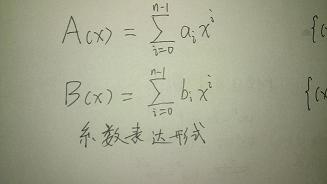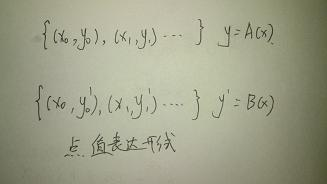加法和乘法：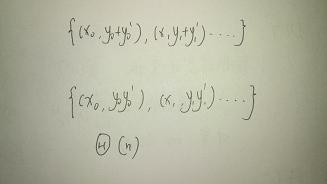B(x)=x^2-x+2  { ( 0 , 2 ) , ( 1 , 2 ) , ( 2 , 4 ) }

C(x)=A(x)+B(x)=2x^2+x+1   { ( 0, 1) , ( 1 , 4 ) , ( 2, 11 ) }

注意乘法需要2n个点 lz比较懒就不写了……

于是我们得到一个计算多项式的方法：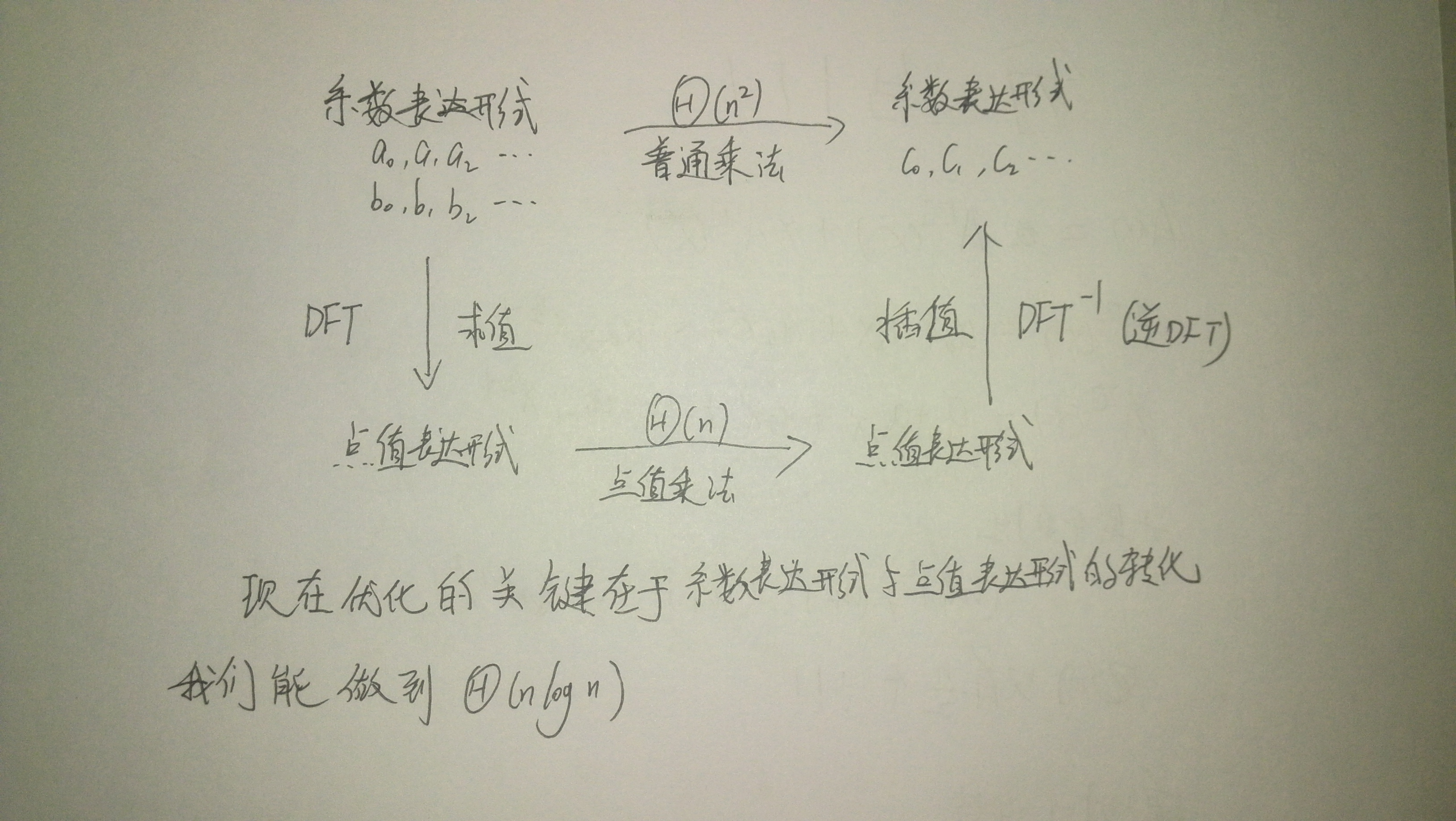2.n次单位复数根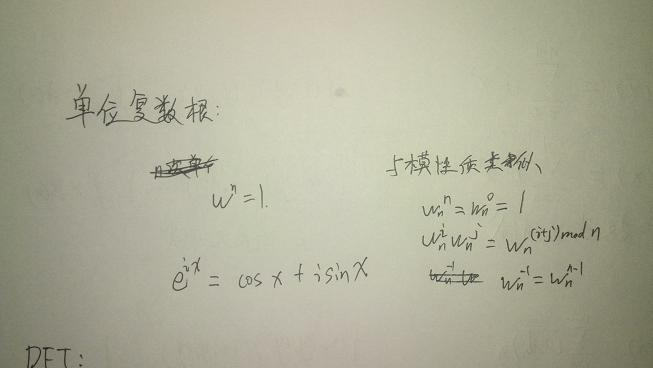有关复数根的性质可以百度到，不再赘述

3.DFT&&FFT

使用单位根计算点值表达式叫DFT（离散傅里叶变换）复杂度n^2，FFT是其优化版复杂度nlogn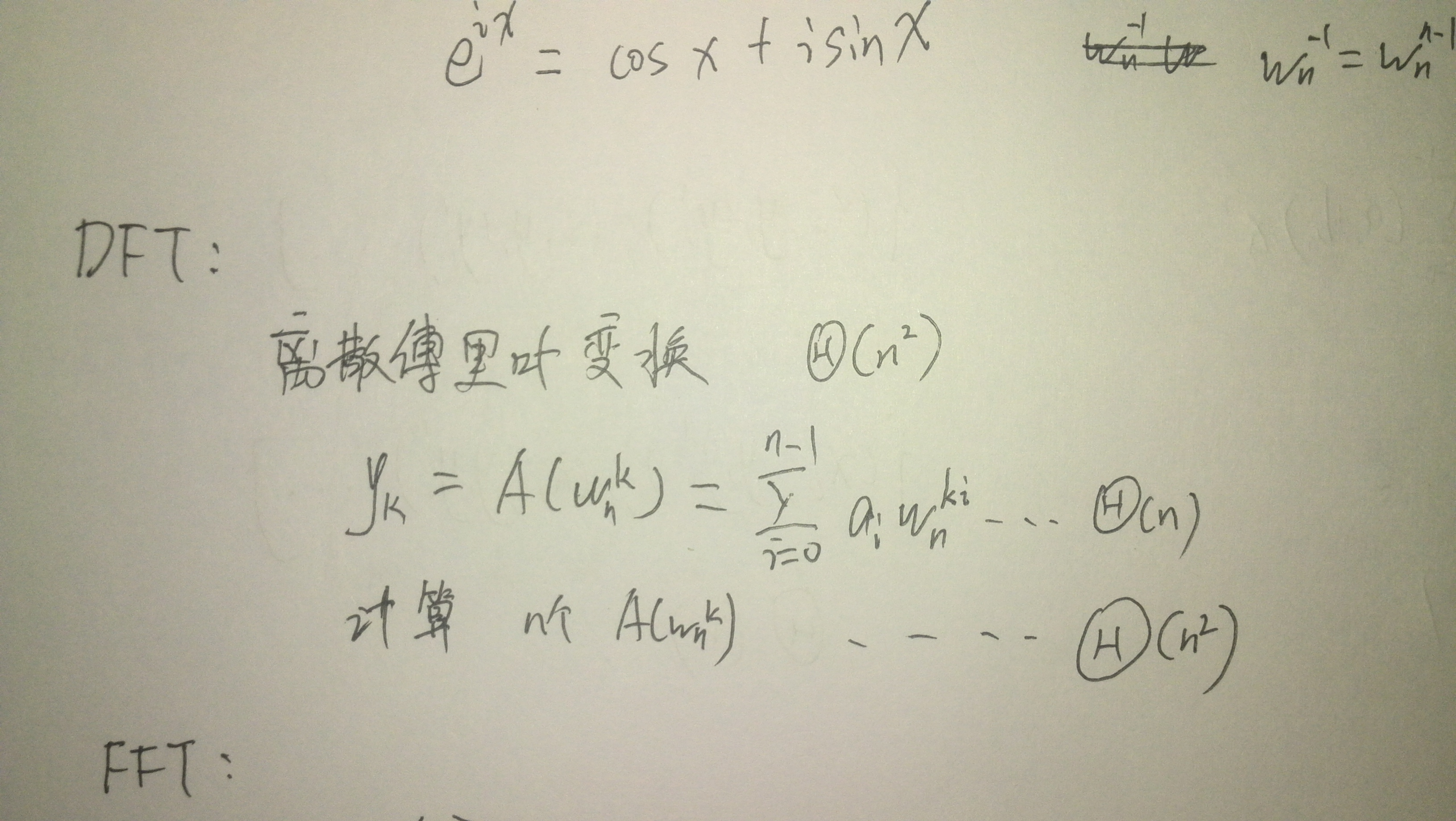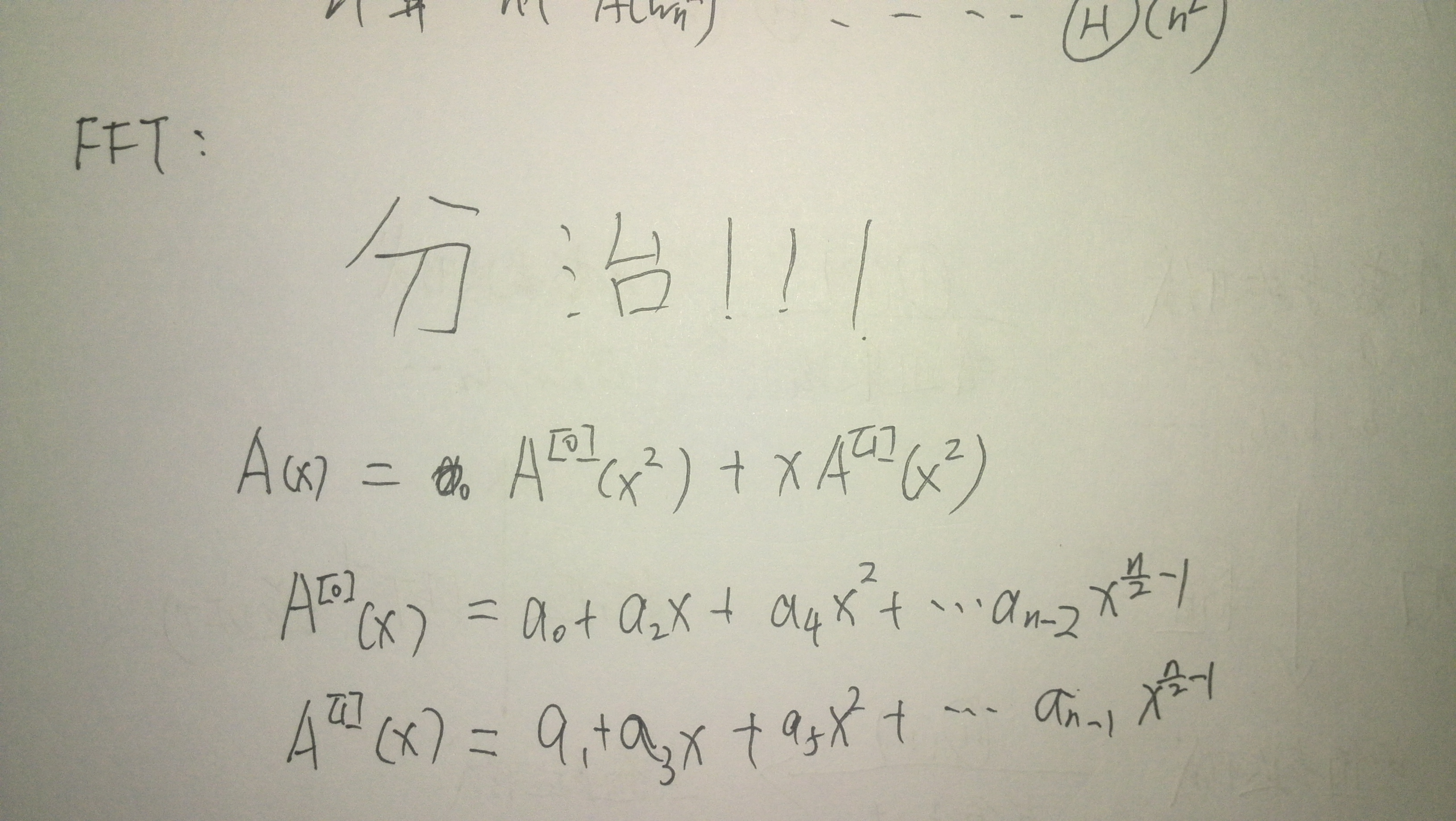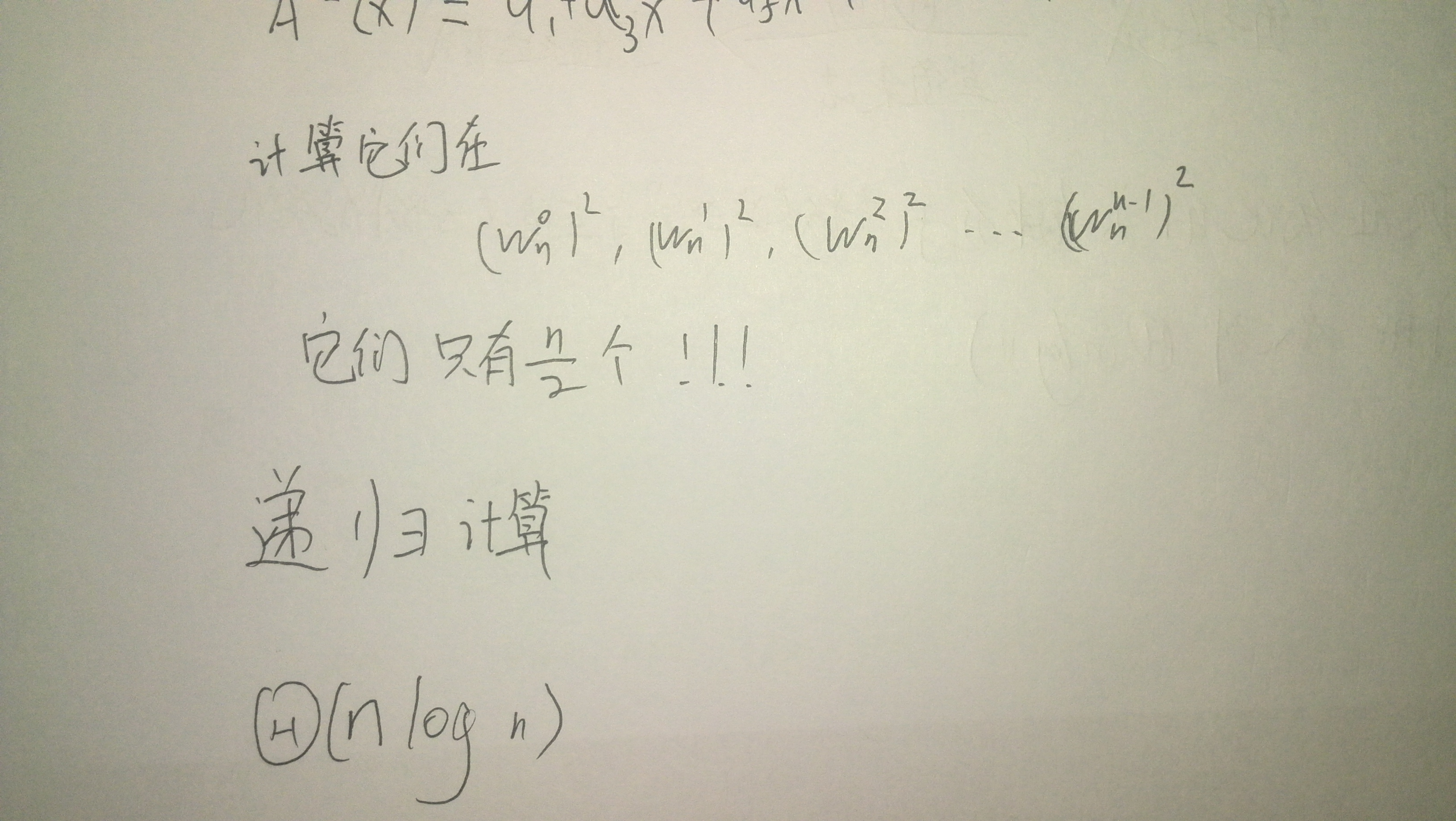计算FFT的伪代码(好吧用的是python)

下划线代表的是下标,括号代表上标，for 循环的range是左闭右开的

[python] view
plain copyFFT(a):
n=a.length()
if n==1:
return a
w_n=e^(pi*i/n)=complex(cos(2*pi/n),sin(2*pi/n))
w=1
a(0)=[a0,a2,...a_n-2]
a(1)=[a1,a3,...a_n-1]
y(0)=FFT(a(0))
y(1)=FFT(a(1))
for k in range(0,n/2):
y_k=y_k(0)+w*y_k(1)
y_k+n/2=y_k(0)-w*y_k(1)
w=w*w_n
return y

4.递归=>迭代??

FFT的for循环中有两次w_n^k*y_k(1)的计算，于是可以改写成这样

[python] view
plain copyfor k in range(0,n/2):
t=w*y_k(1)
y_k=y_k(0)+t
y_k+n/2=y_k(0)-t
w=w*w_n
#这一过程被称蝴蝶操作

观察每次按照奇偶位置分割所形成的树：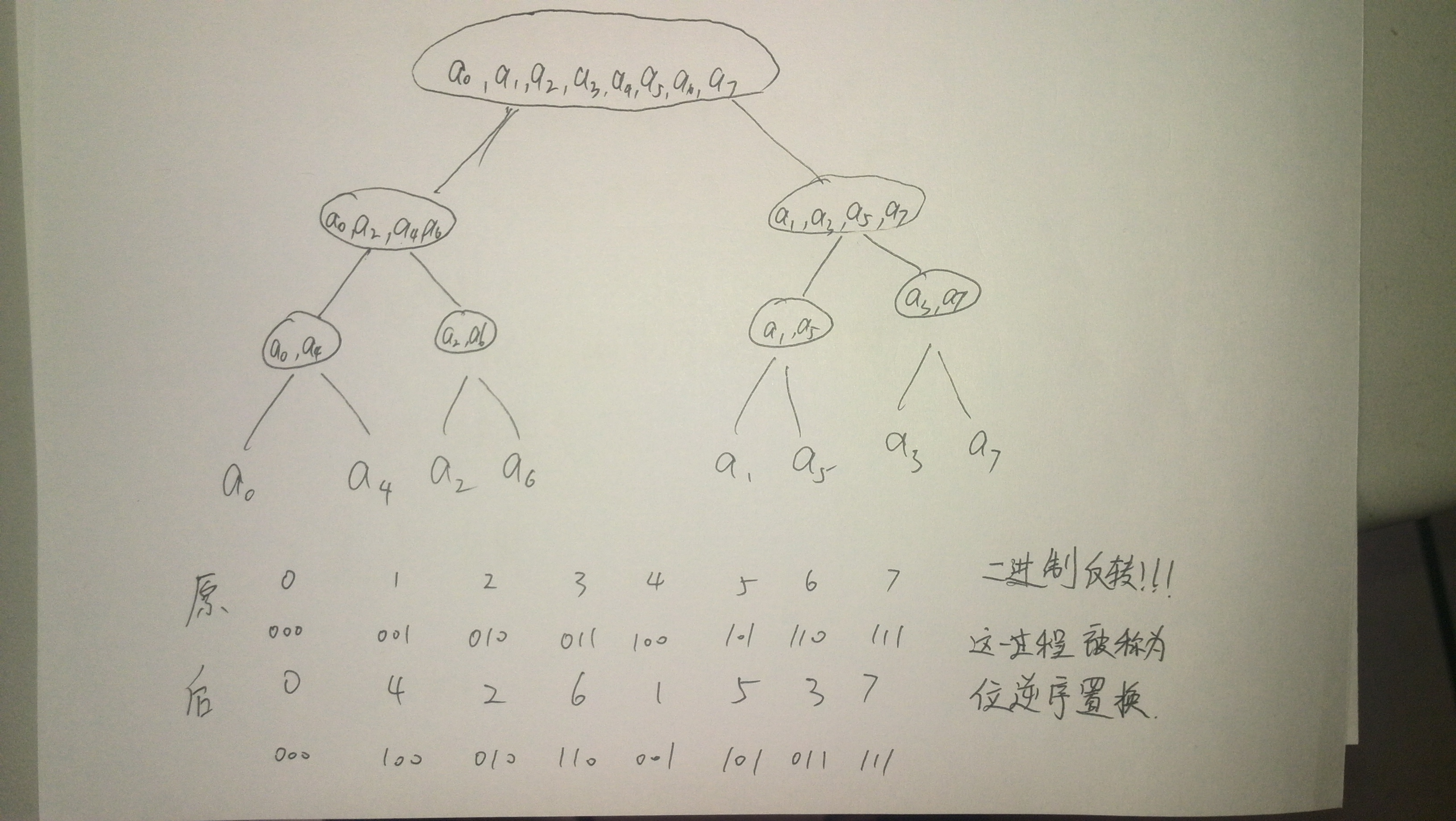每个数和他二进制相反的位置互换！！

伪代码

[python] view
plain copyBIT-REVERSE-COPY(a,A):
n=a.length()
for k in range(0,n):
A[rev(k)]=a_k
#网上说rev函数很好写，就没写……

于是我们给出FFT的迭代实现的伪代码：

[python] view
plain copyFFT(a):
BIT-REVERSE-COPY(a,A)
n=a.length()
for s in range(1,log2(n)+1):
m=2^s
w_m=e^(2*pi*i/m)=complex(cos(2*pi*m),sin(2*pi*m))
for k in range(0,n,m):
w=1
for j in range(0,m/2):
t=w*A[k+j+m/2]
u=A[k+j]
A[k+j]=u+t
A[k+j+m/2]=u-t
w=w*w_m
return A

差不多讲完了,最后给出C++代码

[cpp] view
plain copy#include<bitset>
#include <cstdio>
#include <cstring>
#include <cmath>
#include <algorithm>
#define N 400005
#define pi acos(-1.0) // PI值
using namespace std;
struct complex
{
double r,i;
complex(double real=0.0,double image=0.0){
r=real; i=image;
}
// 以下为三种虚数运算的定义
complex operator + (const complex o){
return complex(r+o.r,i+o.i);
}
complex operator - (const complex o){
return complex(r-o.r,i-o.i);
}
complex operator * (const complex o){
return complex(r*o.r-i*o.i,r*o.i+i*o.r);
}
}x1[N],x2[N];
char a[N/2],b[N/2];
int sum[N]; // 结果存在sum里
int vis[N];
void brc(complex *a,int l){//原来神犇的二进制平摊反转置换太神看不懂，蒟蒻写了一个O(n)的……
memset(vis,0,sizeof(vis));//O(logn)的在后面
for(int i=1;i<l-1;i++){
int x=i,y=0;
int m=(int)log2(l)+0.1;
if(vis[x])continue;
while(m--){
y<<=1;
y|=(x&1);
x>>=1;
}
vis[i]=vis[y]=1;
swap(a[i],a[y]);
}
}
void fft(complex *y,int l,double on) // FFT O(nlogn)
// 其中on==1时为DFT，on==-1为IDFT
{
register int h,i,j,k;
complex u,t;
brc(y,l); // 调用反转置换
for(h=2;h<=l;h<<=1) // 控制层数
{
// 初始化单位复根
complex wn(cos(on*2*pi/h),sin(on*2*pi/h));
for(j=0;j<l;j+=h) // 控制起始下标
{
complex w(1,0); // 初始化螺旋因子
for(k=j;k<j+h/2;k++) // 配对
{
u=y[k];
t=w*y[k+h/2];
y[k]=u+t;
y[k+h/2]=u-t;
w=w*wn; // 更新螺旋因子
} // 据说上面的操作叫蝴蝶操作…
}
}
if(on==-1)  for(i=0;i<l;i++) y[i].r/=l; // IDFT
}
/*
void fft2(complex *a,int s,int t){//蒟蒻自己写的递归版FFT，不保证正确 ,代码内部有未定义变量
if((n>>t)==1)return;//s记录起始,t记录深度,调用时应从0开始
fft(a,s,t+1);
fft(a,s+(1<<t),t+1);
for(int i=0;i<(n>>(t+1));i++){
p=(i<<(t+1))+s;
wt=w[i<<t]*a[p+(1<<t)];
tt[i]=a[p]+wt;
tt[i+(n>>(t+1))]=a[p]-wt;
}
for(i=0;i<(n>>t);i++)a[(i<<t)+s]=tt[i];
}*/
int main(void)
{
int l1,l2,l;
register int i;
while(scanf("%s%s",a,b)!=EOF)
{
l1=strlen(a);
l2=strlen(b);
l=1;
while(l<l1*2 || l<l2*2)   l<<=1; // 将次数界变成2^n
// 配合二分与反转置换
for(i=0;i<l1;i++) // 倒置存入
{
x1[i].r=a[l1-i-1]-'0';
x1[i].i=0.0;
}
for(;i<l;i++)    x1[i].r=x1[i].i=0.0;
// 将多余次数界初始化为0
for(i=0;i<l2;i++)
{
x2[i].r=b[l2-i-1]-'0';
x2[i].i=0.0;
}
for(;i<l;i++)    x2[i].r=x2[i].i=0.0;
fft(x1,l,1); // DFT(a)
fft(x2,l,1); // DFT(b)
for(i=0;i<l;i++) x1[i]=x1[i]*x2[i]; // 点乘结果存入a
fft(x1,l,-1); // IDFT(a*b)
for(i=0;i<l;i++) sum[i]=x1[i].r+0.5; // 四舍五入
for(i=0;i<l;i++) // 进位
{
sum[i+1]+=sum[i]/10;
sum[i]%=10;
}
l=l1+l2-1;
while(sum[l]<=0 && l>0)   l--; // 检索最高位
for(i=l;i>=0;i--)    putchar(sum[i]+'0'); // 倒序输出
putchar('\n');
}
return 0;
}
/*void brc(complex *y,int l) // 二进制平摊反转置换 O(logn)
{
register int i,j,k;
for(i=1,j=l/2;i<l-1;i++)
{
if(i<j)  swap(y[i],y[j]); // 交换互为下标反转的元素
// i<j保证只交换一次
k=l/2;
while(j>=k) // 由最高位检索，遇1变0，遇0变1，跳出
{
j-=k;
k>>=1;
}
if(j<k)  j+=k;
}
}*/

pyc神犇的写法，bzoj3527，zjoi2014 力，无限YM

[cpp] view
plain copy#include<cmath>
#include<cstdio>
#include<cstring>
#include<iostream>
#include<algorithm>
using namespace std;
const int maxn=1000010;
int n,N,L;
int rev[maxn];
int dig[maxn];
double p[maxn];
struct cp{
double r,i;
cp(double _r=0,double _i=0):
r(_r),i(_i){}
cp operator+(cp x){return cp(r+x.r,i+x.i);}
cp operator-(cp x){return cp(r-x.r,i-x.i);}
cp operator*(cp x){return cp(r*x.r-i*x.i,r*x.i+i*x.r);}
};
cp a[maxn],b[maxn],c[maxn],A[maxn],x,y;
void FFT(cp a[],int flag){
for(int i=0;i<N;i++)A[i]=a[rev[i]];
for(int i=0;i<N;i++)a[i]=A[i];
for(int i=2;i<=N;i<<=1){
cp wn(cos(2*M_PI/i),flag*sin(2*M_PI/i));
for(int k=0;k<N;k+=i){
cp w(1,0);
for(int j=0;j<i/2;j++){
x=a[k+j];
y=w*a[k+j+i/2];
a[k+j]=x+y;
a[k+j+i/2]=x-y;
w=w*wn;
}
}
}
if(flag==-1)for(int i=0;i<N;i++)a[i].r/=N;
}
double anss[maxn];
int main(){
scanf("%d",&n);
for(int i=0;i<n;i++)scanf("%lf",&p[i]);
for(L=0,N=1;N<n;N<<=1,L++);L++;N<<=1;
for(int i=0;i<N;i++){
int len=0;
for(int t=i;t;t>>=1)dig[len++]=t&1;
for(int j=0;j<L;j++)rev[i]=rev[i]*2+dig[j];
}
for(int i=0;i<n;i++)a[i]=cp(p[i],0);
for(int i=1;i<n;i++)b[i]=cp(1.0/i/i,0);
FFT(a,1);FFT(b,1);
for(int i=0;i<N;i++)c[i]=a[i]*b[i];
FFT(c,-1);
for(int i=0;i<n;i++)anss[i]=c[i].r;
memset(a,0,sizeof(a));
memset(b,0,sizeof(b));
for(int i=0;i<n;i++)a[i]=cp(p[n-i-1],0);
for(int i=1;i<n;i++)b[i]=cp(1.0/i/i,0);
FFT(a,1);FFT(b,1);
for(int i=0;i<N;i++)c[i]=a[i]*b[i];
FFT(c,-1);
for(int i=0;i<n;i++)anss[i]-=c[n-i-1].r;
for(int i=0;i<n;i++)
printf("%.9f\n",anss[i]);
return 0;
}

重新过了一遍高精乘

[cpp] view
plain copy#include<cstdio>
#include<cmath>
#include<cstring>
#include<iostream>
#include<algorithm>
using namespace std;
const int maxn=1e6+10;
struct cp{
double r,i;
cp(double _r=0,double _i=0):
r(_r),i(_i){}
cp operator+(cp x){return cp(r+x.r,i+x.i);}
cp operator-(cp x){return cp(r-x.r,i-x.i);}
cp operator*(cp x){return cp(r*x.r-i*x.i,r*x.i+i*x.r);}
};
cp a[maxn],b[maxn],A[maxn],x,y,c[maxn];
char s1[maxn],s2[maxn];
int sum[maxn],a1[maxn],a2[maxn],dig[maxn];
int len1,len2,rev[maxn],N,L;
void FFT(cp a[],int flag){
for(int i=0;i<N;i++)A[i]=a[rev[i]];
for(int i=0;i<N;i++)a[i]=A[i];
for(int i=2;i<=N;i<<=1){
cp wn(cos(2*M_PI/i),flag*sin(2*M_PI/i));
for(int k=0;k<N;k+=i){
cp w(1,0);
for(int j=k;j<k+i/2;j++){
x=a[j];
y=a[j+i/2]*w;
a[j]=x+y;
a[j+i/2]=x-y;
w=w*wn;
}
}
}
if(flag==-1)for(int i=0;i<N;i++)a[i].r/=N;
}
int main(){
scanf("%s%s",s1,s2);
len1=strlen(s1);
len2=strlen(s2);
for(N=1,L=0;N<max(len1,len2);N<<=1,L++);N<<=1;L++;
for(int i=0;i<N;i++){
int len=0;
for(int t=i;t;t>>=1)dig[len++]=t&1;
for(int j=0;j<L;j++)rev[i]=(rev[i]<<1)|dig[j];
}
for(int i=0;i<len1;i++)a1[len1-i-1]=s1[i]-'0';
for(int i=0;i<len2;i++)a2[len2-i-1]=s2[i]-'0';
for(int i=0;i<N;i++)a[i]=cp(a1[i]);
for(int i=0;i<N;i++)b[i]=cp(a2[i]);
FFT(a,1);FFT(b,1);
for(int i=0;i<N;i++)c[i]=a[i]*b[i];
FFT(c,-1);
for(int i=0;i<N;i++)sum[i]=c[i].r+0.5;
for(int i=0;i<N;i++){
sum[i+1]+=sum[i]/10;
sum[i]%=10;
}
int l=len1+len2-1;
while(sum[l]==0&&l>0)l--;
for(int i=l;i>=0;i--)
putchar(sum[i]+'0');
putchar('\n');
return 0;
}

查看原文


展开全文• 1.图像的傅里叶频谱的意义之前的博文其实已经归纳过这方面的内容了。我们常用的图像平滑处理，其实就是一个低通滤波，一定程度上去除高频信号，可以使得图像变得柔和（也就是平滑）。但是，在去除周期性噪声时候，...opencv 图像合成 c
•频谱分析
• 注：本文为博主参考书籍和他人文章并加上自己的理解所编，作为学习笔记使用并将其分享出去供大家学习。若涉及到引用您的文章内容请评论区告知！...一、什么是傅里叶变换   时域及频域  在讲...傅里叶变换 傅里叶级数 图像处理 欧拉公式
• 原 如何理解 图像傅里叶变换的频谱图 2018年09月18日 16:43:00 Ring__Rain 阅读数：965 ...
•傅里叶频谱
• 快速傅里叶变换，将其频率零点移至中心并增强显示其傅里叶频谱
• 1、为什么要进行傅里叶变换，其物理意义是什么？ 傅立叶变换是数字信号处理领域一种很重要的算法。要知道傅立叶变换算法的意义，首先要了解傅立叶原理的意义。 傅立叶原理表明：任何连续测量的时序或信号，都可以...
• 图像的傅里叶频谱特性分析 图像傅里叶频谱关于(/，/)的对称性 图像傅里叶频谱特性及其频谱傅里叶变换在图像处理中的应用
• 这是可以取样，然后经过傅里叶变换后显示的频谱图，用C++6.0 写的，达到可视化界面的的效果
• 如何用VC++编写信号频率为30Hz的傅里叶频谱分析程序，并且可以画出频谱图~
• 不过这个说明只是针对一维的傅里叶变换，在图像处理中我们最常见的还是二维频谱，二维频谱到底该怎么看呢？以下是我的理解，谢谢某人的帮助。 1.先看一段MATLAB代码 I = imread('cell.tif'); fI = fft2(I); sfI = ...
• 图像傅立叶频谱分析 参考：http://cns-alumni.bu.edu/~slehar/fourier/fourier.html#filtering 很棒 分析： 如果输入二维图像数据，则显示的图像是输入的灰度分布，傅立叶频谱是输入的频率分布，频谱图中心对称。 ...傅里叶 图像 二维 opencv
• 1、将一幅图分别进行X轴与Y轴上的平移，所得的傅里叶频谱与原图像频谱傅里叶频谱有什么变化，请说明理由。 2、将一幅图进行离散傅里叶变换，得到其傅里叶频谱图，在对原图像进行一定角度的旋转，得到的频谱图与原...哈达玛变换 图像处理 图像拼接 离散余弦变换
• 冈萨雷斯数字图像处理(第三版) matlab代码 图3.5 傅里叶频谱及对数变换matlab代码
• 刚入手傅里叶变化，头大的很，尤其是经过傅里叶变化之后，看频谱，心里慌慌的，不过接触多了，也就明了的差不多了，回头在搜索资料，恨没有早点看到这个资料。 先说收获： 傅里叶变换之后，频谱有几个特点： ...
• 关于傅立叶频谱分析，权威，对于深度了解傅立叶变换有重要意义傅立叶变换
...